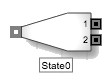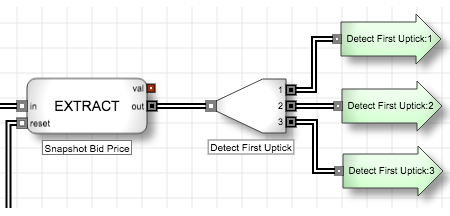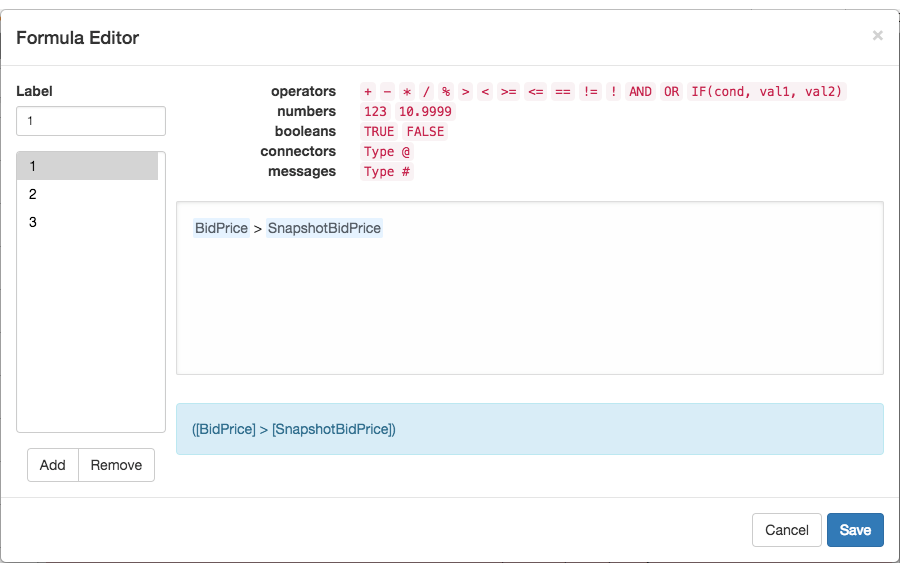Discrete blocks

# State blockThe State block receives an input discrete event message and routes the unchanged message to one of its output ports based on its internal formulas. For each output, you define a formula that evaluates to a Boolean result. When triggered by an incoming discrete event message, the State block begins evaluating its formulas until one of the evaluates to TRUE; then it routes the message through the corresponding output port. By default, the block exposes two discrete event message output ports, but you can add extra ports and define their formulas using the Formula Editor.

When a user-made True/False statement turns TRUE, the State block generates and outputs a discrete event message in the following manner:

• Directs the message to the #1 output if the statement tied to it evaluates to TRUE
• Directs the message to the #2 output if the statement tied to it evaluates to TRUE
• Directs the message each additional port added to the block and outputs if the statement tied to it evaluates to TRUE
• When multiple True/False statements turn TRUE at once, a message outputs from the top-most port.

After outputting its discrete event message, the State block will not re-evaluate its formulas until it receives another incoming discrete event message.

Example The State block uses its internal formulas to define three states. When triggered by the incoming discrete event message, the State block evaluates its formulas and passes the discrete event message through the appropriate port to be used by downstream logic.The Formula Editor for this block defines formulas for each of the three outputs. The formula for the first port uses the > operator to compare the value of the Bid Price (from a Field) block with the value of the Snapshot Bid Price (from the Value Extractor block). The remaining formulas use the = and < operators, respectively.## Block properties

To customize the block, update the Block Properties.

Property Description
Name Name to display beneath the block on the ADL canvas
Formulas

Equations used to calculate the value of the block's output ports

The edit link opens the Formula Editor.